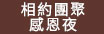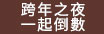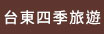• # 縱谷線

熱門景點

•• where are you going?

為你挑選台東縱谷熱景點旅遊路線，幫您規畫路沿途路線及鄰近住宿，現在去台東縱谷旅遊吧!
"; if(!empty(\$txt2)) \$output.="

".\$result["th_col1"]."

"; if(!empty(\$txt3)) \$output.="

".\$result["th_col2"]."

"; if(!empty(\$txt4)) \$output.="

".\$result["th_col3"]."

"; if(!empty(\$txt5)) \$output.="

".\$result["th_col4"]."

"; if(!empty(\$txt6)) \$output.="

".\$result["th_col5"]."

"; if(!empty(\$txt7)) \$output.="

".\$result["th_col6"]."

"; if(!empty(\$txt8)) \$output.="

".\$result["th_col7"]."

"; if(!empty(\$txt9)) \$output.="

".\$result["th_col8"]."

"; \$output.="
"; if(strpos(\$piclink,".swf")!==false) \$output=""; }else{ \$piclink="nopic.jpg"; \$output=""; } if(\$type=="js") \$output="document.write('".addslashes(\$output)."')"; }//end type1 if(\$qty>1){ \$sql="select * from oki_thedata_banner where th_status<>'-1' and th_open='Y' \$sqlcol "; if(!empty(\$cate)) \$sql.=" and th_upid='".\$cate."'"; \$sql.=make_sqlstr_date("th_opendate","th_closedate",\$cfg["date"]); \$sql.=" order by \$order limit 0,\$qty "; \$result=DB_GetAll(\$sql); /* echo ""; */ if(!empty(\$result)){ \$i=0; \$output=""; \$idrand="oki_ad".rand(10000,99999)."_"; foreach(\$result as \$value){ \$link="javascript:body.focus()"; \$linktype=\$value["th_subodr"]; if(!empty(\$value)) \$piclink=\$value["th_detail"]; if(!empty(\$value["th_subremark"])) \$link=\$value["th_subremark"]; \$tags=""; \$tags.=\$value["th_col1"]; \$tags.=\$value["th_col2"]; \$tags.=\$value["th_col3"]; \$tags.=\$value["th_col4"]; \$tags.=\$value["th_col5"]; \$tags.=\$value["th_col6"]; \$tags.=\$value["th_col7"]; \$tags.=\$value["th_col8"]; \$tags.=\$value["th_remark"];//文章那格 \$i++; \$none="none"; if(\$i==1) \$none=""; \$output.="
"; //(strpos(\$piclink,".swf")!==false) ? \$output.="" : \$output.=""; //\$output.="
\n"; (strpos(\$piclink,".swf")!==false) ? \$output.="" : \$output.=""; if(!empty(\$txt)) \$output.="

".\$value["th_title"]."

"; //標題 if(!empty(\$txt1)) \$output.="

".\$value["th_remark"]."

"; //文章 if(!empty(\$txt2)) \$output.="

".\$value["th_col1"]."

"; //縣市 if(!empty(\$txt3)) \$output.="

".\$value["th_col2"]."

"; //鄉鎮 if(!empty(\$txt4)) \$output.="

".\$value["th_col3"]."

"; //類別 if(!empty(\$txt5)) \$output.="

".\$value["th_col4"]."

"; //景點 if(!empty(\$txt6)) \$output.="

".\$value["th_col5"]."

"; //四季 if(!empty(\$txt7)) \$output.="

".\$value["th_col6"]."

"; //活動 if(!empty(\$txt8)) \$output.="".\$value["th_col7"].""; //主題 if(!empty(\$txt9)) \$output.="

".\$value["th_col8"]."

"; //其他 \$output.="
\n"; } \$outputjs=\$idrand."now=1\n"; \$outputjs.=\$idrand."all=\$i\n"; \$outputjs.="function chg_".\$idrand."(){\n"; \$outputjs.="for(i=1;i<=".\$idrand."all;i++){\n"; \$outputjs.="document.getElementById('".\$idrand."'+i).style.display='none'\n"; \$outputjs.="}\n"; \$outputjs.=\$idrand."now++\n"; \$outputjs.="if(".\$idrand."now>".\$idrand."all) ".\$idrand."now=1 \n"; \$outputjs.="document.getElementById('".\$idrand."'+".\$idrand."now).style.display=''\n"; \$outputjs.="}\n"; \$outputjs.="setInterval(chg_".\$idrand.",\$delay)\n"; if(\$show=="all") { \$output=str_replace("","",\$output); \$output=str_replace("display:none","",\$output); \$outputjs =""; } }else{ \$piclink="nopic.jpg"; \$output=""; } if(\$type=="js"){ //\$output="document.write('".addslashes(str_replace("\n","",\$output))."')\n".\$outputjs; //\$output="document.write('".addslashes( \$output )."')"; //\$output=str_replace("\n","');\n document.write('",\$output).\$outputjs; \$rdm_id="oki_pt_".rand(1,3000000); \$opt="document.write('
');\n"; \$opt.="document.getElementById('".\$rdm_id."').innerHTML='".addslashes( str_replace("\n","",\$output) )."'"; \$output=\$opt; }else{ if(!empty(\$outputjs)) \$output.="\n\n"; } } echo \$output; ?>
•• where are you going?

在台東旅行的日子-背起背包，踩著輕躍的步伐，開始屬於自已的旅行吧!

## 延平紅葉村

•••● ◤冬戀蘭陽溫泉季 即將溫暖您的心◢ ● ◤2023 勞工親子暨特色產業嘉年華◢ ● ◤「2023蘭陽媽祖文化節」展開海陸遶境傳承宗教信仰與文化◢ ● ◤「火金姑的故鄉」小礁溪迎接螢光盛宴 語音生態導覽◢ ● ◤我們的小時候 租稅宣導公益園遊會◢標示為"可線上預定"，"使用國旅卡"之商家，無標示之商家請以電話詢問訂房# 台東住宿